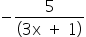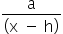Mathematics
Easy

Question

# What is the horizontal asymptote of y =?

## y = -5 y = -3y = 0 y = 3Hint:

## The correct answer is: y = 0

### We have to find the horizontal asymptote of the given graph.We know, For the function, f(x) =+ kHorizontal asymptote: y = k.Vertical asymptote: x = h.Here, value of k is 0 and vertical asymptote: y = k. So, horizontal asymptote of y = -5/(3x+1) is y=0.Hence, the correct option is B.

Horizontal asymptote of a graph is y = k and vertical asymptote is x = h, where (h,k) is a moving point on the graph.

### Related Questions to study#### With Turito Foundation.#### Get an Expert Advice From Turito.